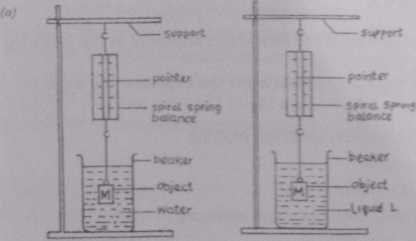Physics Paper 1A, May/June. 2012
 Questions: 1 2 3
Weakness/Remedies
Strength

Question 1A

Question 1Study the diagrams above and use them as guides in carrying out the following instructions

(i) Using the spring balance provided, determine the weight of object of mass M = 50.0g in air Record this weight as W1

(ii)Determine the weight of the object when it iv completely immersed in water contained in a ;beaker at shown in the diagram above Record the weight W2

(iii) Determine the weight of the object when it Is completely immersed in the liquid labelled L Record the weight as W3

(iv)  Evaluate U= (W1-W2) and V= (W1 – W3)

(v)Repeat the procedure with the objects of masses M= 1OOg, 15O g, 200 g and 250 g

(vi)      In each case, evaluate U= (W1-W2) and V= (W1 – W3)

2. Plot a graph with V on the vertical axis and U on the horizontal axis
3. Determine the slopes, of the graph
4. State two precautions taken to ensure accurate results

(b)    (i) State Archimedes' principle
(ii) A piece of brass of mass 20.0g is hung on a spring balance from a rigid support and completely immersed in kerosene of density 8.0 x 102 kgm \ Determine the reading on the spring balance.

_____________________________________________________________________________________________________
observation

This was a popular question and candidates performed very well in it. However, some candidates did not convert L the unit of mass (grammes) into newton. Though some spring balances in the market are calibrated in grammes, so in response to the question, some candidates presented their answers in grammes and it was accommodated by the marking scheme. It was also discovered that some candidates recorded their first set of spring balance readings in air correctly but got the readings in liquid t out of trend. The weights measured (ii air and liquid L, were not probably recorded/tabulated immediately after measurement hence were muddled up.

b (i) This was well stated by most candidates
(ii) The numerical part was not well tackled by most candidates.

(i)    Five values of weight of object in air W1 recorded in gm/IN
(ii)Five values of weight of object in wafer (W2) in gm/N recorded and in trend (Trend: W2 < W1)
(iii)Five values of weight of object in liquid L (W3) to 1 d. p and in trend (Trend W1 > W3 >W2)
(iv)Five values of U =(W1 – W2) correctly evaluated
(v)Five values of V (W1 - W3) correctly evaluated
(vi)Composite table showing W1, W2, w3,  U and V

Candidates are also expected to:
(i)Plot five points on graph
(ii) Distinguish between the axes
(iii)Chose reasonable scales
(iv)Draw line of best fit
(v)Determine the slope of the graph

State any two of the following precautions.

1. Avoided parallax error in reading spring balance
2. Avoided noted zero error of spring balance
3. Avoided splashing of liquid
4. Avoided object touching bottom or sides of beaker
5. Cleaned mass before dipping it into liquid
6.  Repeated readings shown on the table.

(b) (i) Archimedes’ Principle - states that when a body is wholly or partially
immersed in a fluid (liquid) it experiences an upthrust which is equal to the weight of the fluid(liquid) displaced.
(ii) (Weight of Kerosene displaced) * ( volume of object) x (Density of Kerosene) x£
Mass of object x density of kerosene x g Density of object

20 x 10^3 x 8.0 x 10^3 x 10 8x 10^3

0.02N   = Upthrust of kerosene on object
Weight of object in air = Mass x g
20 x 10'3 x 10 = 0.2N
Tension in the spring - (weight of object - Upthrust in air) = 0.2 -0.02
Reading of the balance = 0.18N